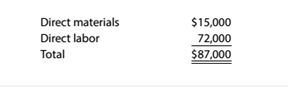Chapter 13, Problem 13.25E

Chapter
Section
Textbook Problem

Direct materials and direct labor variances At the beginning of August, Havasu Primers Company budgeted 30,000 books to be printed in August at standard direct materials and direct labor costs as follows:The standard materials price is $0.40 per pound. The standard direct labor rate is$12 per hour. At the end of August, the actual direct materials and direct labor costs were as follows:There were no direct materials price or direct labor rate variances for August. In addition, assume no changes in the direct materials inventory balances in August. Havasu Printers Company actually produced 24.500 units during August. Determine the direct materials quantity variance, the direct labor time variance, and the total variance.

To determine

Concept Introduction:

Direct material variances:

Direct material variances refer to the difference between the standard direct material cost and actual direct material cost incurred. Direct material cost variances are categorized into following two categories:

1. Direct material Rate variance: this variance shows the difference of standard rate and actual rate of material. The formula to calculate this variance is as follows:
2.   Direct material rate variance = (Actual rate – Standard rate) ×Actual Quantity

3. Direct material usage variance: this variance shows the difference of standard usage and actual usage of material. The formula to calculate this variance is as follows:
4.   Direct material usage variance = (Actual Quantity – Standard Quantity)×Standard rate

5. Direct material cost variance: this variance shows the difference of standard cost and actual cost of material. The formula to calculate this variance is as follows:
6.   Direct material cost variance = Direct material rate variance + Direct material usage variance

Direct labor variances:

Direct labor variances refer to the difference between the standard direct labor cost and actual direct labor cost incurred. Direct labor cost variances are categorized into following categories:

7. Direct labor Rate variance: this variance shows the difference of standard rate and actual rate of labor. The formula to calculate this variance is as follows:
8.   Direct labor rate variance = (Actual rate – Standard rate) ×Actual hours

9. Direct labor efficiency variance: this variance shows the difference of standard usage and actual usage of labor. The formula to calculate this variance is as follows:
10.   Direct labor efficiency variance = (Actual hours – Standard hours)×Standard rate

11. Direct labor cost variance: this variance shows the difference of standard cost and actual cost of labor. The formula to calculate this variance is as follows:
12.   Direct labor cost variance = Direct labor rate variance + Direct labor efficiency variance

Or

Direct Labor cost variance = Actual Labor cost − Standard Labor Cost

To Calculate:

The Direct Material Quantity Variance and Direct Labor time (efficiency) variance.

Explanation

The Direct Material Quantity Variance and Direct Labor time (efficiency) variance are calculated as follows:

 Direct Material Quantity Variance: Actual Quantity (A) (13220/$0.40) 33050 Lbs. Standard Quantity (B) (15000/$0.40) 37500 Lbs. Standard Price (C) $0.40 Per lbs. Direct Material Quantity Variance (D)= (A-B)*C =$ 1,780...

Still sussing out bartleby?

Check out a sample textbook solution.

See a sample solution

The Solution to Your Study Problems

Bartleby provides explanations to thousands of textbook problems written by our experts, many with advanced degrees!

Get Started

Find more solutions based on key concepts